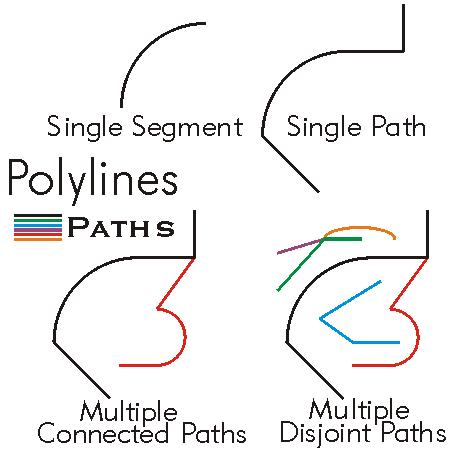This document is archived and information here might be outdated.  Recommended version.

IPolyline Interface (ArcObjects .NET 10.8 SDK)
 ArcObjects Help for .NET developers > ArcObjects Help for .NET developers > ArcObjects namespaces > Geometry > ESRI.ArcGIS.Geometry > Interfaces > IP > IPolyline Interface
 ArcGIS Developer Help

# IPolyline Interface

#### Product Availability

Available with ArcGIS Engine, ArcGIS Desktop, and ArcGIS Server.

#### Description

A polyline is a collection of one or many paths.  The paths do not have to be connected to each other.  A polyline can be defined either as a PointCollection, SegmentCollection, or GeometryCollection.  Each collection type allows the user to access and manipulate different aspects of the polyline geometry.

#### Members

Name DescriptionDensify Converts this polycurve into a piecewise linear approximation of itself.Dimension The topological dimension of this geometry.Envelope Creates a copy of this geometry's envelope and returns it.FromPoint The 'from' point of the curve.Generalize Generalizes this polycurve using the Douglas-Peucker algorithm.GeometryType The type of this geometry.GeoNormalize Shifts longitudes, if need be, into a continuous range of 360 degrees.GeoNormalizeFromLongitude Normalizes longitudes into a continuous range containing the longitude. This method is obsolete.GetSubcurve Extracts a portion of this curve into a new curve.IsClosed Indicates if 'from' and 'to' points (of each part) are identical.IsEmpty Indicates whether this geometry contains any points.Length The length of the curve.Project Projects this geometry into a new spatial reference.QueryEnvelope Copies this geometry's envelope properties into the specified envelope.QueryFromPoint Copies this curve's 'from' point to the input point.QueryNormal Constructs a line normal to a curve from a point at a specified distance along the curve.QueryPoint Copies to outPoint the properties of a point on the curve at a specified distance from the beginning of the curve.QueryPointAndDistance Finds the point on the curve closest to inPoint, then copies that point to outPoint; optionally calculates related items.QueryTangent Constructs a line tangent to a curve from a point at a specified distance along the curve.QueryToPoint Copies the curve's 'to' point into the input point.Reshape Modifies this polyline by replacing some of its segments with some segments from reshapeSource.ReverseOrientation Reverses the parameterization of the curve ('from' point becomes 'to' point, first segment becomes last segment, etc).SetEmpty Removes all points from this geometry.SimplifyNetwork Removes zero length segments (zero in 2 dimensions), merges parts at endpoints that only connect to each other, reorients segments that are pointing against the prevailing orientation for a part.Smooth Converts this curve into a smooth curve containing only Bezier curve segments.SnapToSpatialReference Moves points of this geometry so that they can be represented in the precision of the geometry's associated spatial reference system.SpatialReference The spatial reference associated with this geometry.SplitAtDistance Introduces a new vertex into this polyline at a specified distance from the beginning of the polyline.SplitAtPoint Introduces a new vertex into this polyline at the location on it closest to the input point.ToPoint The 'to' point of the curve.Weed Generalizes using a small tolerance based upon either the system units of the geometry's spatial reference, or the geometry's bounding box.

#### Inherited Interfaces

Interfaces Description
IPolycurve Provides access to members that define operations common to polylines and the boundaries of polygons.
ICurve Provides access to properties and methods of all 1 dimensional curves (polylines, segments, boundaries of polygons, etc.).
IGeometry Provides access to members that describe properties and behavior of all geometric objects.

#### Classes that implement IPolyline

Classes Description
Polyline An ordered collection of paths; optionally has measure, height and ID attributes.

#### Remarks

To define a polyline containing many disjoint paths, the polyline must be defined as a GeometryCollection to which preconstructed path geometries are added.  Adding segments to a polyline defined as a SegmentCollection, or points to a polyline defined as a PointCollection, assumes that the polyline is a single path of connected segments.